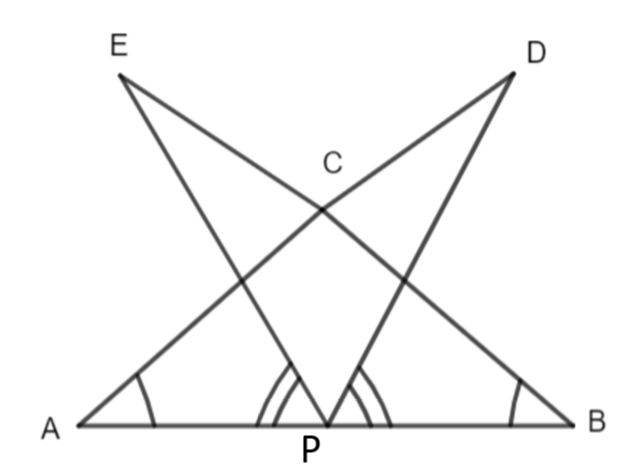Courses
Courses for Kids
Free study material
Free LIVE classes
MoreLIVE
Join Vedantu’s FREE Mastercalss

# AB is a line segment and P is its midpoint. D and E are the points on the same side of AB such that $\angle BAD=\angle ABE$ and $\angle EPA=\angle DPB.$ Show that $\left( a \right)\Delta DAP\cong \Delta EBP$$\left( b \right)AD=DE$Verified
335.1k+ views
Hint: First, we will show that $\Delta DAP\cong \Delta EBP.$ We will see that we have two angles and one side between them which allows the SAS congruence rule to predict the congruence of triangles DAP and EBP. Then, we will find AD = BE as they are corresponding parts of the congruent triangle.

Complete step-by-step solution:
Here, we are given that,
$\angle BAD=\angle ABE.......\left( i \right)$
$\angle EPA=\angle DPB.....\left( ii \right)$
As P is the midpoint of AB, we can say,
$AP=PB......\left( iii \right)$
$\left( a \right)\Delta DAP\cong \Delta EBP$
Here, we have to show that triangle DAP is congruent to triangle EBP. Now, from (ii), we know that,
$\angle EPA=\angle DPB$
Adding angle EPD to both sides, we get,
$\angle EPA+\angle EPD=\angle DPB+\angle EPD$
$\angle DPA=\angle EPB......\left( iv \right)$
In triangle DAP and triangle EBP, we have,
$\angle DPA=\angle EPB\left[ \text{From (iv)} \right]$
$AP=BP\left[ \text{As P is the midpoint} \right]$
$\angle PAD=\angle PBE\left[ \text{As }\angle BAD=\angle ABE\text{ given} \right]$
So, by SAS congruence rule, we get,
$\Delta DAP\cong \Delta EBP$
Hence, we have proved that triangle DAP is congruent to triangle EBP.
We have proved (a) that $\Delta DAP\cong \Delta EBP.$ We know that congruent triangles have their corresponding parts equal.
So, in $\Delta DAP\cong \Delta EBP,$ AD is corresponding to BE.
Note: Students need to remember that $\angle EPA=\angle DPB$ will not be considered a part in proving the congruence for triangle DAP and triangle EBP because those angles are not a full part of the triangle and for that, we have to first add angle EPD to both sides. Also, we can see that angles BAD and ABE are equal which implies that angle PAD is equal to angle PBE since P lies on the same line. Students should not use angle BAD equal to angle ABE in the proof of congruence as it has not been part of the triangle DAP and triangle EBP, we need to use angle PAD is equal to angle PBE.